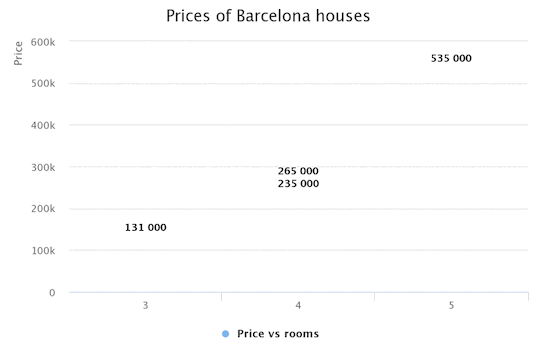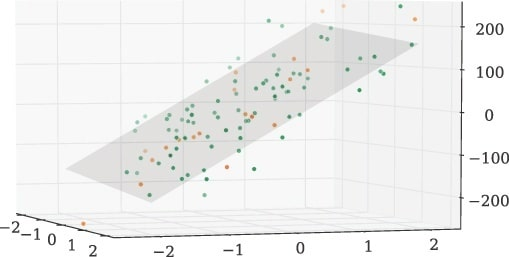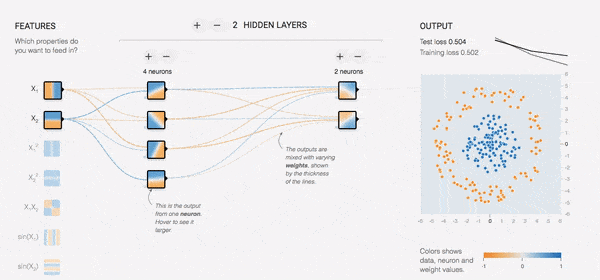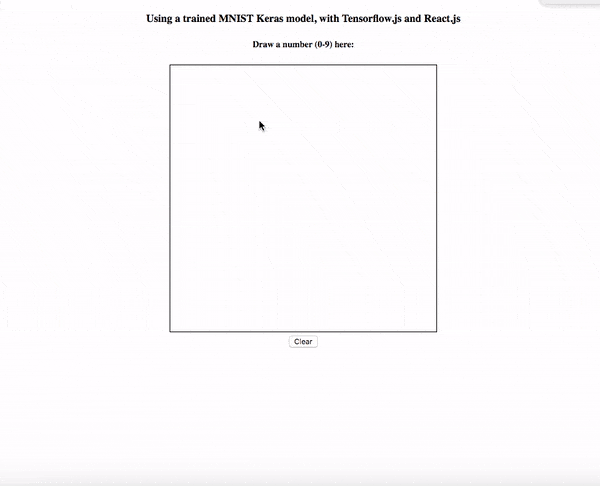# First steps with TensorFlow.js

I would like to do more articles explaining a little bit about all the machine learning and deep learning basics. I'm a beginner in this area, but I'd like to explain soon these concepts to create some interesting AI models. Nevertheless, we don't need a deep knowledge about machine learning to use some existing models. We can use some libraries like Keras, Tensorflow or TensorFlow.js. We are going to see here how to create basic AI models and use more sophisticated models with TensorFlow.js. Although it's not required a deep knowledge, we are going to explain few concepts.

## What is a Model?

Or maybe a better question would be: 'What is the reality?'. Yes, that's quite complex to answer... We need to simplify it in order to understand it! A way to represent a part of this simplified "reality"  is using a model. So; there are infinity kind of models: world maps, diagrams, etc.It's easier to understand the models that we can use without machine help. For example, if we want to do a model to represent the price of Barcelona houses regarding the size of the house: First, we can collect some data:

Number of rooms Prices
3 131.000€
3 125.000€
4 235.000€
4 265.000€
5 535.000€

Then, we display this data on a 2D graph, 1 dimension for each param (price, rooms):And... voilà! We can now draw a line and start predicting some prices of houses with 6, 7 or more rooms. This model is named linear regression and it's one of the most simple models to start in the machine learning world. Of course this model is not good enough:

1. There are only 5 examples so it's not reliable enough.
2. There are only 2 params (price, rooms), yet there are more factors that could have an effect on the price: district, the age of the house, etc.

For the first problem, we can deal with it by adding more examples, e. g. 1.000.000 examples instead of 5. For the second problem, we can add more dimensions... right? With 2D chart we can understand the data and draw a line while in 3D dimensions we could also use a plane:But, how to deal with more than 3D? 4D or 1000000D? Our mind can't visualize this on a chart but... good news! We can use maths and calculate hyperplanes in more than 3D and neural networks are a great tool for this! By the way, I have good news for you; using TensorFlow.js you don't need to be a math expert.

## What is a neural network?

Before understanding what is a neural network, we need to know what is a neuron. A neuron, in the real world looks similar to this:The most important parts of a neuron are:

• Dendrites: It's the input of the data.
• Axon: It's the output.
• Synapse (not in the image): It's the structure that permits a neuron to communicate with another neuron. It is responsible to pass electric signals between the nerve ending of the axon and a dendrite of a near neuron. These synapses are the key to learn because they increase or decrease the electrical activity depending on the usage.

A neuron in machine learning (simplified):• Inputs: The parameters of the input.
• Weights: Like synapses, their activity increase or decrease to adjust the neuron in order to establish a better linear regression.
• Linear function: Each neuron is like a linear regression function so for a linear regression model we only need one neuron!
• Activation function: We can apply some activation function to change the output from a scalar to another non-linear function. The more common; sigmoid, RELU and tanh.
• Output: The computed output after applying the activation function.

The usage of an activation function is very useful, it's the power of a neural network. Without any activation function it's not possible to have a smart neuron network. The reason is that although you have multiple neurons in your network, the output of the neural network is always going to be a linear regression. We need some mechanism to deform this individual linear regressions to be non-linear to solve the non-linear problems. Thanks to activation functions we can transform these linear functions to non-linear functions:## Training a model

Drawing a line in our chart, as in the 2D linear regression example, is enough for us to start predicting new data. Nevertheless, the idea of "deep learning" is that our neural network learn to write this line. For a simple line we can use a very simple neural network with only one neuron, but for another models maybe we want to do more complex things like classify two groups of data. In this case, the "training" is going to learn how to draw something like this:Remember that this is not complex because it's in 2D. Every model is a world, but the concept of "training" is very similar in all of them. The first step is drawing a random line, and improving it in a iteration algorithm, fixing the error in each iteration. This optimization algorithm has the name of Gradient Descent (there are more sophisticated algorithms as SGD or ADAM, with the same concept). In order to understand the Gradient Descent, we need to know that every algorithm (linear regressor, logistic regressor, etc.) has a different cost function to measure this error. The cost functions always converge in some point and can be convex and non-convex functions. The lowest converge point is found on the 0% error. Our aim is to achieve this point.When we work with the Gradient Descent algorithm, we start in some random point of this cost function but, we don't know where is it! Imagine that your are on the mountains, completely blind, and you need to walk down, step by step, to the lowest point. If the land is irregular (like non-convex functions), the descent is going to be more complex.I'm not going to explain Gradient Descent algorithm deeply. Just remember that it's the optimization algorithm to train the AI models to minimize the error of predictions. This algorithm requires time and GPU for matrix multiplications. This converge point is usually hard to achieve in the first execution so we need to fix some hyperparameters like the learning rate (size of the step down the hill) or add some regularization. After the iterations of Gradient Descent we get a closer point to the converge point when the error is close to 0%. At this moment, we already have the model created and we are ready to start predicting!## Training a model with TensorFlow.js

TensorFlow.js provides us with an easy way to create neural networks. At first, we are going to create a LinearModel class with a method trainModel. For this kind of model we are going to use a sequential model. A sequential model is any model where the outputs of one layer are the inputs to the next layer, i.e. when the model topology is a simple 'stack' of layers, with no branching or skipping. Inside the method trainModel we are going to define the layers (we are going to use only one because it's enough for a Linear Regression problem):

``````import * as tf from '@tensorflow/tfjs';

/**
* Linear model class
*/
export default class LinearModel {
/**
* Train model
*/
async trainModel(xs, ys){
const layers = tf.layers.dense({
units: 1, // Dimensionality of the output space
inputShape: , // Only one param
});
const lossAndOptimizer = {
loss: 'meanSquaredError',
optimizer: 'sgd', // Stochastic gradient descent
};

this.linearModel = tf.sequential();
this.linearModel.compile(lossAndOptimizer);

// Start the model training!
await this.linearModel.fit(
tf.tensor1d(xs),
tf.tensor1d(ys),
);
}

...more
}``````

To use this class:

``````const model = new LinearModel()

// xs and ys -> array of numbers (x-axis and y-axis)
await model.trainModel(xs, ys)``````

After this training, we are ready to start predicting!

## Predicting with TensorFlow.js

Predicting normally is the easier part! Training a model requires to define some hyperparameters... but still, predicting is so simple. We are going to write the next method into the LinearRegressor class:

``````import * as tf from '@tensorflow/tfjs';

export default class LinearModel {
...trainingCode

predict(value){
return Array.from(
this.linearModel
.predict(tf.tensor2d([value], [1, 1]))
.dataSync()
)
}
}``````

Now, we can use the prediction method in our code:

``````const prediction = model.predict(500) // Predict for the number 500
console.log(prediction) // => 420.423``````You can play with the code here:

## Use pre-trained models with TensorFlow.js

Learning to create models is the most difficult part; normalizing the data for training, deciding all the hyperparams correctly,  etc.  If you are a beginner in this area (like me) and you want to play with some models, you can use pre-trained models. There are a lot of pre-trained models that you can use with TensorFlow.js. Moreover, you can import external models, created with TensorFlow or Keras. For example, you can use the posenet model (Real-time human pose estimations) for funny projects:📕 Code: https://github.com/aralroca/posenet-d3 It's very easy to use:

``````import * as posenet from '@tensorflow-models/posenet'

// Constants
const imageScaleFactor = 0.5
const outputStride = 16
const flipHorizontal = true
const weight = 0.5

// Do predictions
const poses = await net.estimateSinglePose(
imageElement,
imageScaleFactor,
flipHorizontal,
outputStride
)``````

poses variable is this JSON:

``````{
"score": 0.32371445304906,
"keypoints": [
{
"position": {
"y": 76.291801452637,
"x": 253.36747741699
},
"part": "nose",
"score": 0.99539834260941
},
{
"position": {
"y": 71.10383605957,
"x": 253.54365539551
},
"part": "leftEye",
"score": 0.98781454563141
}
// ...And for: rightEye, leftEar, rightEar, leftShoulder, rightShoulder
// leftElbow, rightElbow, leftWrist, rightWrist, leftHip, rightHip,
// leftKnee, rightKnee, leftAnkle, rightAnkle
]
}``````

Imagine how many funny projects you can develop only with this model!## Importing models from Keras

We can import external models into TensorFlow.js. In this example, we are going to use a Keras model for number recognition (h5 file format). For this, we need the tfjs_converter.

``pip install tensorflowjs``

Then, use the converter:

``tensorflowjs_converter --input_format keras keras/cnn.h5 src/assets``

Finally, you are ready to import the model into your JS code!

``````// Load model

// Prepare image
let img = tf.fromPixels(imageData, 1)
img = img.reshape([1, 28, 28, 1])
img = tf.cast(img, 'float32')

// Predict
const output = model.predict(img)``````

Few lines of code is enough to enjoy with the number recognition model from Keras into our JS code. Of course, now we can add more logic into this code to do something more useful, like a canvas to draw a number and then capture this image to predict the number.## Why in the browser?

Training models in the browser can be very inefficient depending on the device. Even thought TensorFlow.js takes advantage of WebGL to train the model behind the scenes, it is 1.5-2x slower than TensorFlow Python. However, before TensorFlow.js, it was impossible to use machine learning models directly in the browser without an API interaction. Now we can train and use models offline in our applications. Also, predictions are much faster because they don't require the request to the server. Another benefit is the low cost in server because now all these calculations are on client-side.## Conclusion

• A model is a way to represent a simplified part of the reality and we can use it to predict things.
• A good way to create models is using neural networks.
• A good and easy tool to create neural networks is TensorFlow.js.